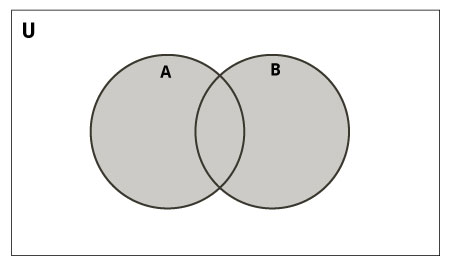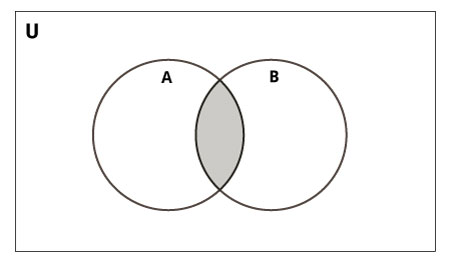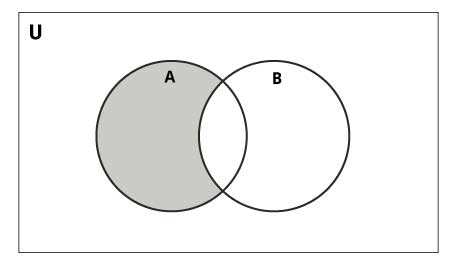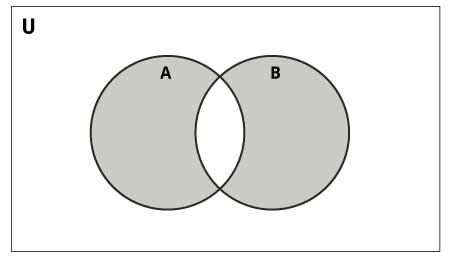# Python Sets

## What is a set in Python?

A set is an unordered collection of items. Every element is unique (no duplicates) and must be immutable (which cannot be changed).

However, the set itself is mutable. We can add or remove items from it.

Sets can be used to perform mathematical set operations like union, intersection, symmetric difference etc.

## How to create a set?

A set is created by placing all the items (elements) inside curly braces {}, separated by comma or by using the built-in function `set()`.

It can have any number of items and they may be of different types (integer, float, tuple, string etc.). But a set cannot have a mutable element, like list, set or dictionary, as its element.

# set of integers
my_set = {1, 2, 3}
print(my_set)
# set of mixed datatypes
my_set = {1.0, "Hello", (1, 2, 3)}
print(my_set)

Try the following examples as well.

# set do not have duplicates
# Output: {1, 2, 3, 4}
my_set = {1,2,3,4,3,2}
print(my_set)
# set cannot have mutable items
# here [3, 4] is a mutable list
# If you uncomment line #12,
# this will cause an error.
# TypeError: unhashable type: 'list'
#my_set = {1, 2, [3, 4]}
# we can make set from a list
# Output: {1, 2, 3}
my_set = set([1,2,3,2])
print(my_set)

Creating an empty set is a bit tricky.

Empty curly braces {} will make an empty dictionary in Python. To make a set without any elements we use the `set()` function without any argument.

## How to change a set in Python?

Sets are mutable. But since they are unordered, indexing have no meaning.

We cannot access or change an element of set using indexing or slicing. Set does not support it.

We can add single element using the `add()` method and multiple elements using the `update()` method. The `update()` method can take tuples, lists, strings or other sets as its argument. In all cases, duplicates are avoided.

# initialize my_set
my_set = {1,3}
print(my_set)
# if you uncomment line 9,
# you will get an error
# TypeError: 'set' object does not support indexing
#my_set
# add an element
# Output: {1, 2, 3}
print(my_set)
# add multiple elements
# Output: {1, 2, 3, 4}
my_set.update([2,3,4])
print(my_set)
# add list and set
# Output: {1, 2, 3, 4, 5, 6, 8}
my_set.update([4,5], {1,6,8})
print(my_set)

When you run the program, the output will be:

```{1, 3}
{1, 2, 3}
{1, 2, 3, 4}
{1, 2, 3, 4, 5, 6, 8}
```

## How to remove elements from a set?

A particular item can be removed from set using methods, `discard()` and `remove()`.

The only difference between the two is that, while using `discard()` if the item does not exist in the set, it remains unchanged. But `remove()` will raise an error in such condition.

The following example will illustrate this.

# initialize my_set
my_set = {1, 3, 4, 5, 6}
print(my_set)
# discard an element
# Output: {1, 3, 5, 6}
print(my_set)
# remove an element
# Output: {1, 3, 5}
my_set.remove(6)
print(my_set)
# discard an element
# not present in my_set
# Output: {1, 3, 5}
print(my_set)
# remove an element
# not present in my_set
# If you uncomment line 27,
# you will get an error.
# Output: KeyError: 2
#my_set.remove(2)

Set being unordered, there is no way of determining which item will be popped. It is completely arbitrary.

We can also remove all items from a set using `clear()`.

# initialize my_set
# Output: set of unique elements
my_set = set("HelloWorld")
print(my_set)
# pop an element
# Output: random element
print(my_set.pop())
# pop another element
# Output: random element
my_set.pop()
print(my_set)
# clear my_set
#Output: set()
my_set.clear()
print(my_set)

## Python Set Operations

Sets can be used to carry out mathematical set operations like union, intersection, difference and symmetric difference. We can do this with operators or methods.

Let us consider the following two sets for the following operations.

``````>>> A = {1, 2, 3, 4, 5}
>>> B = {4, 5, 6, 7, 8}``````

###Union of A and B is a set of all elements from both sets.

Union is performed using `|` operator. Same can be accomplished using the method `union()`.

# initialize A and B
A = {1, 2, 3, 4, 5}
B = {4, 5, 6, 7, 8}
# use | operator
# Output: {1, 2, 3, 4, 5, 6, 7, 8}
print(A | B)
``````
# use union function
>>> A.union(B)
{1, 2, 3, 4, 5, 6, 7, 8}

# use union function on B
>>> B.union(A)
{1, 2, 3, 4, 5, 6, 7, 8}
``````

### Set IntersectionIntersection of A and B is a set of elements that are common in both sets.

Intersection is performed using `&` operator. Same can be accomplished using the method `intersection()`.

# initialize A and B
A = {1, 2, 3, 4, 5}
B = {4, 5, 6, 7, 8}
# use & operator
# Output: {4, 5}
print(A & B)
``````
# use intersection function on A
>>> A.intersection(B)
{4, 5}

# use intersection function on B
>>> B.intersection(A)
{4, 5}
``````

### Set DifferenceDifference of A and B (A - B) is a set of elements that are only in A but not in B. Similarly, B - A is a set of element in B but not in A.

Difference is performed using `-` operator. Same can be accomplished using the method `difference()`.

# initialize A and B
A = {1, 2, 3, 4, 5}
B = {4, 5, 6, 7, 8}
# use - operator on A
# Output: {1, 2, 3}
print(A - B)
``````
# use difference function on A
>>> A.difference(B)
{1, 2, 3}

# use - operator on B
>>> B - A
{8, 6, 7}

# use difference function on B
>>> B.difference(A)
{8, 6, 7}
``````

### Set Symmetric DifferenceSymmetric Difference of A and B is a set of elements in both A and B except those that are common in both.

Symmetric difference is performed using `^` operator. Same can be accomplished using the method `symmetric_difference()`.

# initialize A and B
A = {1, 2, 3, 4, 5}
B = {4, 5, 6, 7, 8}
# use ^ operator
# Output: {1, 2, 3, 6, 7, 8}
print(A ^ B)

Try the following examples on Python shell.

``````
# use symmetric_difference function on A
>>> A.symmetric_difference(B)
{1, 2, 3, 6, 7, 8}

# use symmetric_difference function on B
>>> B.symmetric_difference(A)
{1, 2, 3, 6, 7, 8}``````

## Different Python Set Methods

There are many set methods, some of which we have already used above. Here is a list of all the methods that are available with set objects.

Python Set Methods
Method Description
add() Adds an element to the set
clear() Removes all elements from the set
copy() Returns a copy of the set
difference() Returns the difference of two or more sets as a new set
difference_update() Removes all elements of another set from this set
discard() Removes an element from the set if it is a member. (Do nothing if the element is not in set)
intersection() Returns the intersection of two sets as a new set
intersection_update() Updates the set with the intersection of itself and another
isdisjoint() Returns `True` if two sets have a null intersection
issubset() Returns `True` if another set contains this set
issuperset() Returns `True` if this set contains another set
pop() Removes and returns an arbitary set element. Raise `KeyError` if the set is empty
remove() Removes an element from the set. If the element is not a member, raise a `KeyError`
symmetric_difference() Returns the symmetric difference of two sets as a new set
symmetric_difference_update() Updates a set with the symmetric difference of itself and another
union() Returns the union of sets in a new set
update() Updates the set with the union of itself and others

## Other Set Operations

### Set Membership Test

We can test if an item exists in a set or not, using the keyword `in.`

# initialize my_set
my_set = set("apple")
# check if 'a' is present
# Output: True
print('a' in my_set)
# check if 'p' is present
# Output: False
print('p' not in my_set)

### Iterating Through a Set

Using a for loop, we can iterate though each item in a set.

``````>>> for letter in set("apple"):
...     print(letter)
...    ``````

### Built-in Functions with Set

Built-in functions like `all()``any()``enumerate()``len()``max()``min()``sorted()``sum()` etc. are commonly used with set to perform different tasks.

Built-in Functions with Set
Function Description
all() Return `True` if all elements of the set are true (or if the set is empty).
any() Return `True` if any element of the set is true. If the set is empty, return `False.`
enumerate() Return an enumerate object. It contains the index and value of all the items of set as a pair.
len() Return the length (the number of items) in the set.
max() Return the largest item in the set.
min() Return the smallest item in the set.
sorted() Return a new sorted list from elements in the set(does not sort the set itself).
sum() Retrun the sum of all elements in the set.

## Python Frozenset

Frozenset is a new class that has the characteristics of a set, but its elements cannot be changed once assigned. While tuples are immutable lists, frozensets are immutable sets.

Sets being mutable are unhashable, so they can't be used as dictionary keys. On the other hand, frozensets are hashable and can be used as keys to a dictionary.

Frozensets can be created using the function frozenset().

This datatype supports methods like `copy()``difference()``intersection()``isdisjoint()``issubset()``issuperset()``symmetric_difference()` and `union()`. Being immutable it does not have method that add or remove elements.

# initialize A and B
A = frozenset([1, 2, 3, 4])
B = frozenset([3, 4, 5, 6])

Try these examples on Python shell.

``````>>> A.isdisjoint(B)
False
>>> A.difference(B)
frozenset({1, 2})
>>> A | B
frozenset({1, 2, 3, 4, 5, 6})
...
AttributeError: 'frozenset' object has no attribute 'add'``````
###### #####################################################

s={1,2,3,4}
t={6,7,3}
x={3,7,6}

print(s)

# Itrerator
for ele in s:
print(ele)

print(s)

print(s)

# Union
print(s|t)

# Intersection
print(s&t)

# Set Equal
print(s==t)

#
print(x==t)

# Set Difference
print(s-t)

# Super Set
print(t>=x)

# sub Set
print(t<x)

######################################